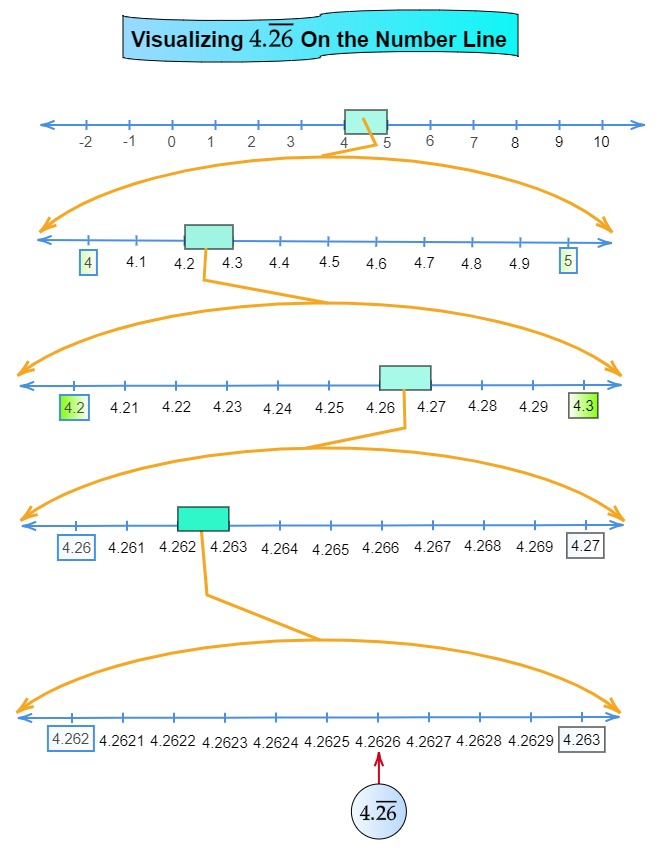# Visualise $4 . \overline{26}$ on the number line, up to 4 decimal places.

Given:

Given number is $4 . \overline{26}$.
To do:

We have to visualise the representation of $4 . \overline{26}$ on the number line using successive magnification.
Solution:

$4 . \overline{26}$ up to 4 decimal places is 4.2626.

Therefore,

$4.2626$ lies between 4 and 5.

So, we divide the number line into 10 equal parts and mark each point of division. The first mark on the right of 4 will be $4.1$ followed by $4.2$ and so on.

The point left of 5 will be $4.9$.

The magnified view of the above will show that $4.2626$ lies between 4.2 and 4.3.

Divide the line segment between 4.2 and 4.3 into 10 equal parts.

The first part will be 4.21 followed by 4.22  and so on.

The magnified view of the above will show that $4.2626$ lies between 4.26 and 4.27.

Divide the line segment between 4.262 and 4.263 into 10 equal parts.

The first part will be 4.2621 followed by 4.2622 and so on.

The magnified view of the above will show that $4.2626$ lies between 4.262 and 4.263 as shown in the below figure.Updated on: 10-Oct-2022

48 Views